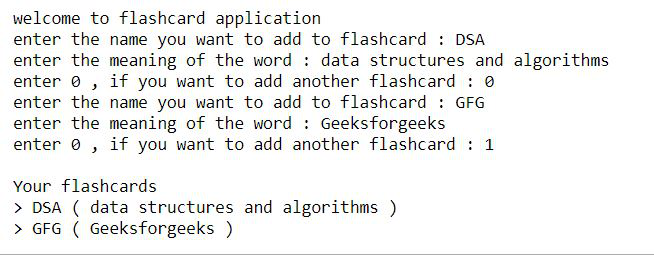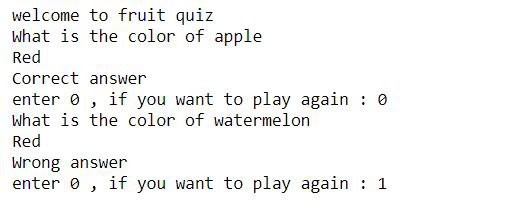Open in App
Not now

# Python program to build flashcard using class in Python

• Difficulty Level : Easy
• Last Updated : 20 Jun, 2022

In this article, we will see how to build a flashcard using class in python. A flashcard is a card having information on both sides, which can be used as an aid in memoization. Flashcards usually have a question on one side and an answer on the other. Particularly in this article, we are going to create flashcards that will be having a word and its meaning.

Let’s see some examples of flashcard:

Example 1:

Approach :

• Take the word and its meaning as input from the user.
• Create a class named flashcard, use the __init__() function to assign values for Word and Meaning.
• Now we use the __str__() function to return a string that contains the word and meaning.
• Store the returned strings in a list named flash.
• Use a while loop to print all the stored flashcards.

Below is the full implementation:

## Python3

 `class` `flashcard:` `    ``def` `__init__(``self``, word, meaning):` `        ``self``.word ``=` `word` `        ``self``.meaning ``=` `meaning` `    ``def` `__str__(``self``):` `      `  `        ``#we will return a string ` `        ``return` `self``.word``+``' ( '``+``self``.meaning``+``' )'` `      `  `flash ``=` `[]` `print``(``"welcome to flashcard application"``)`   `#the following loop will be repeated until` `#user stops to add the flashcards` `while``(``True``):` `    ``word ``=` `input``(``"enter the name you want to add to flashcard : "``)` `    ``meaning ``=` `input``(``"enter the meaning of the word : "``)` `    `  `    ``flash.append(flashcard(word, meaning))` `    ``option ``=` `int``(``input``(``"enter 0 , if you want to add another flashcard : "``))` `    `  `    ``if``(option):` `        ``break` `        `  `# printing all the flashcards ` `print``(``"\nYour flashcards"``)` `for` `i ``in` `flash:` `    ``print``(``">"``, i)`

Output:Example 2:

Approach :

• Create a class named flashcard.
• Initialize dictionary fruits using __init__() method.
• Now randomly choose a pair from fruits using choice() method and store the key in variable fruit and value in variable color.
• Now prompt the user to answer the color of the randomly chosen fruit.
• If correct print correct else print wrong.

## Python3

 `import` `random`   `class` `flashcard:` `    ``def` `__init__(``self``):` `      `  `        ``self``.fruits``=``{``'apple'``:``'red'``,` `                     ``'orange'``:``'orange'``,` `                     ``'watermelon'``:``'green'``,` `                     ``'banana'``:``'yellow'``}` `        `  `    ``def` `quiz(``self``):` `        ``while` `(``True``):` `          `  `            ``fruit, color ``=` `random.choice(``list``(``self``.fruits.items()))` `            `  `            ``print``(``"What is the color of {}"``.``format``(fruit))` `            ``user_answer ``=` `input``()` `            `  `            ``if``(user_answer.lower() ``=``=` `color):` `                ``print``(``"Correct answer"``)` `            ``else``:` `                ``print``(``"Wrong answer"``)` `                `  `            ``option ``=` `int``(``input``(``"enter 0 , if you want to play again : "``))` `            ``if` `(option):` `                ``break`   `print``(``"welcome to fruit quiz "``)` `fc``=``flashcard()` `fc.quiz()`

Output:My Personal Notes arrow_drop_up
Related Articles